# Precalculus : Terms in a Series

## Example Questions

### Example Question #1 : Terms In A Series

Consider the sequence: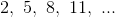What is the fifteenth term in the sequence?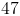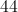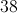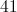Explanation:

The sequence can be described by the equation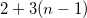, whereis the term in the sequence.

For the 15th term,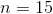.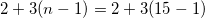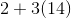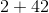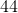### Example Question #1 : Terms In A Series

What is the sum of the firstterms of an arithmetic series if the first term is, and the last term is?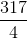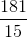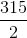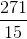Explanation:

Write the formula to find the arithmetic sum of a series whereis the number of terms,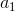is the first term, and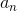is the last term.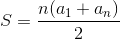Substitute the given values and solve for the sum.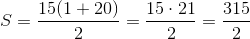### Example Question #1 : Terms In A Series

Given the terms of the sequence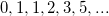, what are the next two terms after?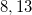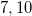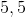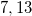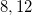Explanation:

The next two terms areand. This is the Fibonacci sequence where you start off with the termsand, and the next term is the sum of two previous terms. So then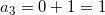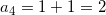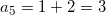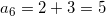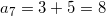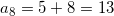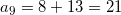and so on.

### Example Question #1 : Terms In A Series

What is the fifth term of the series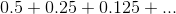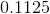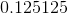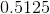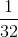Explanation:

Let's try to see if this series is a geometric series.

We can divide adjacent terms to try and discover a multiplicative factor.

Doing this it seems the series proceeds with a common multiple ofbetween each term.

Rewriting the series we get,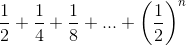.

When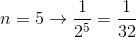.

### All Precalculus Resources